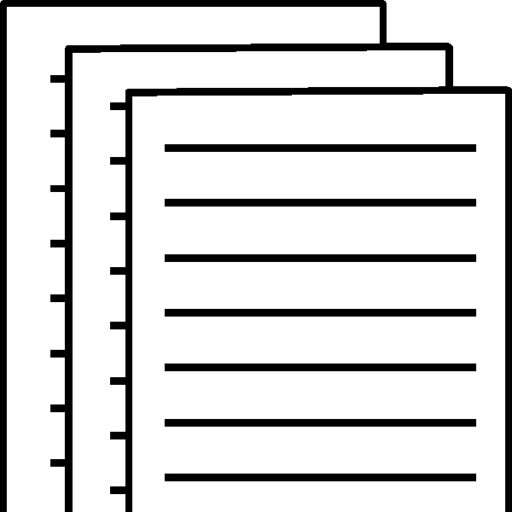## FIN 534 week 2 assignment 1 FIN 534 week 2 assignment 1

NAME

Fin 534

Free cash flow (FCF) represents the cash that a company is able to generate after laying out the money required to maintain or expand its asset base.

1. What is the free cash flow for 2014?

DEBIT (1-Tax rate) +Depreciation-change in net working capital-capital expenditure
158,560(1-.4) +116,960-(-24558)-0 =95136+116960-24558 =187538
Working capital for 2013= current assets –current liabilities= 642400
working capital for 2014=617842

Net working capital= -24558

2. Suppose Congress changed the tax laws so that Berndt’s depreciation expenses doubled. No changes in operations occurred. What would happen to reported profit and to net cash flow?

If the depreciation expense is doubled net income will decrease and cash flow will increase.

Decrease in Net Income=change depreciation amount (1-Tax rate)

120,000(.60)=72,000

Increase in Net Cash Flow=

120,000(.40)=\$48,000

3. Calculate the 2013 current and quick ratios based on the projected balance sheet and income statement data. What can you say about the company’s liquidity position in 2014?

Current Ratio= current assets / current liabilities = 2,680,112/1,039,800=2.57

Quick Ratio= (current asset – inventory) / (current liabilities) =

2,680,112-1,716,480/1,039,800=.93

The company has current ratio of greater than 1 indicating that the firm has decent liquidity and has sufficient level of current assets to meet its current liabilities. The quick ratio is stricter measure of liquidity and a ratio of .93 is an indicator that the firm does not have adequate level of liquid current assets to meet its short term debt obligations and its substantial portion of current assets is in the form of inventory

4. Calculate the 2014 -inventory turnover, days sales outstanding (DSO), fixed assets turnover,andtotal assets turnover.

Inventory turnover = sales / inventory = 4.09

7,035,600/1,716,480=4.09

Days sales outstanding = DSO= account receivable / (total credit sales * number of days ) = 45.5

878,000(7,035,600/365)=45.5 days

Fixed assets turnover = sales / net PPE = 8.40

7,035,600/836,840/ =8.40

Total assets turnover = sales / total assets = 2

7,035,600/3,516,952 = 2

5. Calculate the 2014 debt ratio, liabilities-to-assets ratio, times-interest-earned, and EBITDAcoverage ratios. What can you conclude from these ratios?

Debit ratio = total debt / total assets = 0.22

500,000+300,000/3,516,952 =0.22

Liabilities to assets ratio = total liability/total assets

1,039,800+500,000/3,516,952 = .44

Times interest earned = EBIT/Interest Expense =6.28

502,640/80,000= 6.28

EBITDA coverage ratio = (EBITDA+ Lease Payments)/ Interest Expense+ Lease Payments

502,960+40,000/80,000+40,000 =4.52

6. Calculate the 2014 profit margin, basic earning power (BEP), return on assets (ROA), and returnon equity (ROE). What can you say about these ratios?

Profit Margin Percentage BEP= Net income/ Sales = 0.4

253,584/7,035,600 =.4
Break-even point = EBIT/Total Assets = BEP= .14

502,640/3,516,952=.14

ROA= Return of Assets= Net Income / Average total assets= .07

253,584/3,516,952 =.07

ROE= Return of Equity = Annual Net Income / Average stockholders’ equity =13%

253,584/1,977,152 =.13 %

7.Calculate the 2014 price/earnings ratio, price/cash flow ratio, and market/book ratio.

Price/Earnings ratio = 12.17 / 1.104 = 11.02

Price to cash flow = 12.17 / ((1.104 + 120,000) / 500,000) = 50.7

Market to book ratio = 12.17 / 7.909 = 1.54

8.Use the extended DuPont equation to provide a summary and overview of company’s financial condition as projected for 2014. What are the firm’s major strengths and weaknesses?

ROE = Net Profit * Asset Turnover * Equity Multiplier

Net Profit = 0.04%

Asset Turnover = 2.00

Equity Multiplier = 3,516,952 / 1,977,152 = 1.78

Company’s ROE is positive mainly due to the net profit ratio. If the net profit ratio had been negative the company’s ROE would have been negative. Even though the company reports a positive profit margin it would still be considered as a weakness because of its low, 0.04%, profit margin. The company displays a favorable Asset turnover ratio of 2.00, which would be considered one of the company’s strength. Equity multiplier is a double-edged sword as it magnifies the profit and loss for the shareholder. Under the current circumstances when company is struggling to generate enough profit it would be considered as weakness of the company.

To view and download a complete answer, scroll down to the bottom to pay